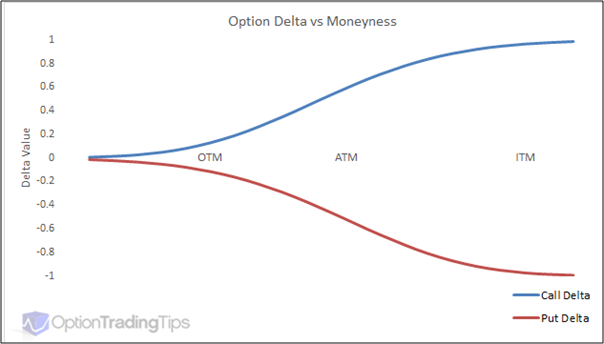What do we understand by Option Delta and how do we apply it - Motilal Oswal
What do we understand by Option Delta and how do we apply it - Motilal Oswal

# What do we understand by Option Delta and how do we apply it?If you are trading options then you must have come across the term called Option Greeks. Options Greeks are second level analysis of options which enable a more in depth analysis of options to take smarter positions in options. One such measure is Options delta. So, how to calculate option delta? What is option delta as a concept and what are its applications? How to use option delta in practice?

What exactly is option delta?
Option delta is a measure of sensitivity of the price of an option to the changes in the price of the underlying. For example if we are looking at a Reliance 960 call option expiring on the last Thursday of March 2018 then the option delta will measure how the option price of this particular contract moves with respect to changes in the price of Reliance spot in the cash market. Delta is one of the four major analytical risk measures when it comes to options. The option delta of a call option will vary from 0 to 1 while the option delta of a put option will vary from 0 to -1.

Even within the call option, the delta will be the highest for an in-the-money call option which will be closer to 1 while it will be closer to 0 in case of out-of-the-money call option. Effectively, call options will have a positive delta while put options will have a negative delta. Why do ITM options have a higher delta compared to OTM options? Let us take the case of Reliance Industries again. Let us assume that the spot price of Reliance in the cash market is Rs.940. Let us also assume that an ITM Reliance 920 call option is quoting at Rs.28 when the spot price of Reliance is Rs.940. Out of this Rs.28 option premium, Rs.20 is represented by intrinsic value and Rs.8 represents time value. When the price of RIL moves up to 960 then the intrinsic value increases and so does the time value. On the contrary in case of an OTM 980 call, even after the price of Reliance goes up to Rs.960, it is still out of the money. So the only movement is in terms of time value and that is why the impact is much lower in case of OTM options. That explains why the OTM options have a lower delta compared to ITM options.

How to interpret the Delta number for a call option?
Different traders use different scales for measuring option delta. While some use a scale of 0 to 1, others use a scale of 0-100. So a delta value of 0.60 will be equivalent to 60 in the other scale, but the underlying concept remains the same. So what does a delta of 0.60 really mean? It shows the sensitivity which means that if the price of the underlying moves up Rs.20 then the price of the call option with a delta of 0.6 will by Rs.12. Let us look at the illustrative table below when the spot price of Reliance is Rs.940..

Particulars910 call920 call930 call940  call950 call960 callMoneynessITMITMITMATMOTMOTMDelta+0.70+0.60+0.55+0.50+0.30+0.25Option price453220853RIL spot Price shiftRs.20Rs.20Rs.20Rs.20Rs.20Rs.20Impact on optionRs.14Rs.12Rs.11Rs.10Rs.6Rs.5

As we can see in the above RIL call option, as we move from ITM to OTM options the delta keeps going down and thus the impact on the option price for every unit change in the price of RIL also keeps going down.
The chart below captures the option delta movement for calls and puts based on whether they are ATM, ITM or OTM..As can be seen from the above chart, option delta of a put is exactly the mirror image of the option delta of a call. Typically, deep ITM and deep OTM calls and puts do not really have a substantial impact on delta shifts. The impact of delta shifts is the maximum around the ATM strike.

Four things you need to know about option delta

Delta is also the probability of the option expiring in the money (ITM). Which is why an ATM has delta of around 0.5, deep ITM options have delta closer to 1 and deep OTM options have delta closer to 0.

Delta is positive for call options and negative for put options. That is because a rise in price of the stock is positive for call options while it is negative for put options.

Delta is not constant and keeps changing over a period of time. Delta depends on factors like volatility, interest rates and time to maturity.

The sign of the delta is the direction indicator. A positive delta means that you are long on the market and a negative delta means that you are short on the market.

Finally, a word on delta hedging
Delta hedging is commonly used to reduce the risk associated with price movements in the underlying asset. Delta hedging actually helps us to offset long and short positions. If the trader holds one call option with a delta of 0.50 and one put option with a delta of -0.50 then the net delta of the position is 0. Typically, straddles have a zero delta. Delta hedging can also be done with stocks and options. How does it work? Let us say you are holding a call option with a delta of 0.70. If the lot size of the stock is 100 shares then you can perfectly hedge 1 lot of the call option by selling 700 shares of the stock. Conversely, if you are long on a put option with delta of 0.70 then you can hedge you position perfectly by buying 700 shares of the stock. That is the crux of delta hedging!